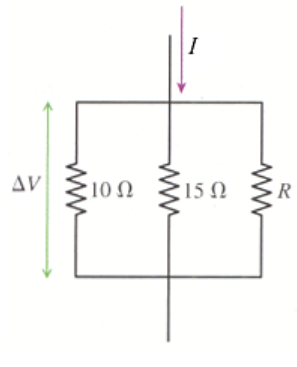# Problem: What is the value of resistor R in the figure below, in which I = 8 A and ΔV = 34 V?

###### FREE Expert Solution

Equivalent resistance in parallel resistors:

$\overline{)\frac{\mathbf{1}}{{\mathbf{R}}_{\mathbf{e}\mathbf{q}}}{\mathbf{=}}\frac{\mathbf{1}}{{\mathbf{R}}_{\mathbf{1}}}{\mathbf{+}}\frac{\mathbf{1}}{{\mathbf{R}}_{\mathbf{2}}}{\mathbf{+}}\frac{\mathbf{1}}{{\mathbf{R}}_{\mathbf{3}}}}$

Ohm's law:

$\overline{){\mathbf{V}}{\mathbf{=}}{\mathbf{i}}{\mathbf{R}}}$

Req = (1/10 + 1/15 + 1/R)-1 = (1/6 + 1/R)-1 = 6R/(R + 6)###### Problem Details

What is the value of resistor R in the figure below, in which I = 8 A and ΔV = 34 V?